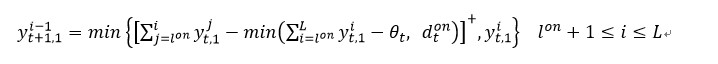•Gurobi Staff
Hi Aldo,

You see this error because you are trying to sum over Gurobi general expressions (GenExpr). The error traces back to the part below:
sum(max_((beta - (stocks @ ret[i])), 0) for i in range(T))

The GenExpr are temporary objects and do not have any member functions such as addition or subtraction.

We suggest defining a new decision variable $$y = (\beta - x^T \hat{r}^t)^+$$. This allows to define your objective function in terms of $$y$$ and then add constraints to enforce the relationship between $$y$$ and $$x$$.

Please be aware that you will see an error if having:
y = model.addMVar(T, name="y")model.addConstrs(     (y[i] == max_((beta - (stocks @ ret[i])), 0) for i in range(T)), name="set_max")
The main reason is the left and the right hand sides do not have the right types (see below copied from the documentation). General constraint helper functions like max_() are not currently integrated with matrix variable objects.
• Min or Max Constraint: an expression of the form x == op_(y), where x is a Var object, and y is a Var, a list of Var and constants, or a tupledict of Var, and op_ is one of min_ or max_.

If you want to continue using GenExpr, you can use MVar.tolist() to map MVar objects to Var objects.

y = model.addMVar(T, lb = -GRB.INFINITY, name="y")z = model.addVars(T, name="z")y_list = y.tolist()model.addConstrs(    (y[i] == beta - (stocks @ ret[i]) for i in range(T)), name="map_to_aux")model.addConstrs(    (z[i] == max_(y_list[i], 0) for i in range(T)), name="set_max")

Best regards,

Maliheh

•i encounter a similar problem. the constriant is :and my code is:

for t in range(T):
for i in range(l_on+1, L):
m.addConstr(y[t+1,0,i-1] == min_( max_( gp.quicksum(y[t,0,j] for j in range(l_on-1, i+1)) - min_( gp.quicksum(y[t,0,k] for k in range(l_on-1,L)) - theta, d_on[t] ), 0), y[t,0,i]+0))

the error is:

TypeError: unsupported operand type(s) for *: 'int' and 'GenExpr'

•Gurobi Staff

Hi Yin,

The error traces back to the part below in your snippet because you are multiplying the integer -1 by a GenExpr.

- min_(gp.quicksum(y[t,0,k] for k in range(l_on-1,L)) - theta, d_on[t])

That being said, this is not the only issue with your snippet.

• You cannot model general expressions in a nested fashion as you already did. The y in an expression of the form x == op_(y) should be a Var, a list of Var and constants, or a tupledict of Var. It cannot be a GenExpr.
• You need to define a separate auxiliary variable for each of the expressions below:
1) gp.quicksum(y[t, 0, k] for k in range(l_on - 1, L)) - theta)2) min_(gp.quicksum(y[t,0,k] for k in range(l_on-1,L)) - theta, d_on[t])3) gp.quicksum(y[t,0,j] for j in range(l_on-1, i+1)) - min_(gp.quicksum(y[t,0,k] for k in range(l_on-1,L)) - theta, d_on[t])4) max_(gp.quicksum(y[t,0,j] for j in range(l_on-1, i+1)) - min_(gp.quicksum(y[t,0,k] for k in range(l_on-1,L)) - theta, d_on[t]), 0)

The snippet below shows in detail how to do this.

x_1 = m.addVars(T, name="x_1")x_2 = m.addVars(T, name="x_2")x_3 = m.addVars(T, L, name="x_3")x_4 = m.addVars(T, L, name="x_4")for t in range(T):    m.addConstr(x_1[t] == gp.quicksum(y[t, 0, k] for k in range(l_on - 1, L)) - theta)    m.addConstr(x_2[t] == gp.min_(x_1[t], d_on[t]))        for i in range(l_on + 1, L):        m.addConstr(            x_3[t, i]            == gp.quicksum(y[t, 0, j] for j in range(l_on - 1, i + 1)) - x_2[t]        )        m.addConstr(x_4[t, i] == gp.max_(x_3[t, i], 0))        m.addConstr(            y[t + 1, 0, i - 1]            == gp.min_(                  x_4[t, i],                  y[t, 0, i],            )       )
As a side note, in your formula $$\theta$$ depends on $$t$$, however, in the code snippet, it is a scalar constant. You might want to double check this.

Best regards,
Maliheh
•Hi Maliheh，

It works. Thank you so much. You've made my day good one!

Have a GREAT day!

Best regards,
Yin